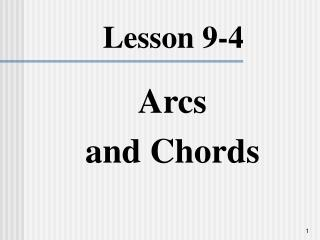Download PresentationLesson 9-4

# Lesson 9-4 - PowerPoint PPT PresentationDownload Presentation## Lesson 9-4

- - - - - - - - - - - - - - - - - - - - - - - - - - - E N D - - - - - - - - - - - - - - - - - - - - - - - - - - -
##### Presentation Transcript

1. Arcs and Chords Lesson 9-4

2. A B E C D Theorem #1: In a circle, if two chords are congruent then their corresponding minor arcs are congruent. Example:

3. D B A E C Theorem #2: In a circle, if a diameter (or radius) is perpendicular to a chord, then it bisects the chord and its arc. Example: If AB = 5 cm, find AE.

4. D F C O B A E Theorem #3: In a circle, two chords are congruent if and only if they are equidistant from the center. Example: If AB = 5 cm, find CD. Since AB = CD, CD = 5 cm.

5. 15cm A B D 8cm O Try Some: • Draw a circle with a chord that is 15 inches long and 8 inches from the center of the circle. • Draw a radius so that it forms a right triangle. • How could you find the length of the radius? Solution: ∆ODB is a right triangle and x

6. A B 10 cm 10 cm O C 20cm D Try Some Sketches: • Draw a circle with a diameter that is 20 cm long. • Draw another chord (parallel to the diameter) that is 14cm long. • Find the distance from the smaller chord to the center of the circle. Solution: ∆EOB is a right triangle. OB (radius) = 10 cm 14 cm E x 7.1 cm